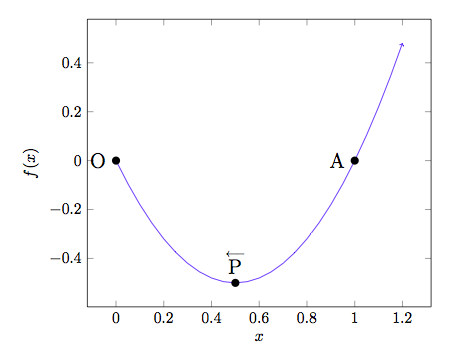# Limiting Angle

Calculus Level 4$A(1, 0)$ is a point on the parabola $y=2x(x-1)$. From point $A$, point $P$ is moving along the curve towards the origin $O(0, 0)$. What is the limit $\lim_{P \rightarrow O } \sec \angle APO ?$

×